Speed of Slovakian trains

Rudolf decided to take the train from the station 'Trnava' to 'Zemianske Kostoľany'. In the train timetables found train R 725 Remata :

km
0Bratislava hl.st.12:57
19Pezinok13:1213:13
46Trnava13:3013:32
63Leopoldov13:4514:01
68Hlohovec14:0614:07
87Zbehy14:2214:23
114Topoľčany14:4914:50
124Chynorany15:0215:04
131Partizánske15:1415:16
145Zemianske Kostoľany15:3215:33
149Nováky15:3815:39
158Prievidza15:49

Calculate the average speed between these stations, travel distance and time.

Result

s =  99 km
t =  2 h
v =  49.5 km/h

Solution:Leave us a comment of this math problem and its solution (i.e. if it is still somewhat unclear...):Be the first to comment!To solve this verbal math problem are needed these knowledge from mathematics:

Looking for help with calculating arithmetic mean? For Basic calculations in analytic geometry is helpful line slope calculator. From coordinates of two points in the plane it calculate slope, normal and parametric line equation(s), slope, directional angle, direction vector, the length of segment, intersections the coordinate axes etc. Looking for a statistical calculator? Do you want to convert length units?

Next similar math problems:

1. Skier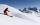At this point, the first skier lead 20 km before the second skier and travels at a constant speed 19 km/h. The second skier rides at 24 km/h. How long take him to catch up the first?
2. Double-track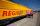On double-track express met with a freight train. Express travel at speed 72 km/h, a freight train at speed 36 km/h. After what time period will be from 9 km apart?
3. Bob traveled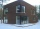Bob traveled 20 meters to Dave's house. He arrived in 3 minutes. What was his average speed for the trip.
4. Cyclist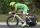A cyclist is moving at 35 km/h and follow pedestrian who is walking at speed 4 km/h. Walker has an advantage 19 km. How long take cyclist to catch up with him?
5. Two cars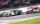Car A1 goes at average speed of 106 km/h and the second car A2 goes at 103 km/h. How many second will it take car A1 to circulate car A2? Assume that both cars are 5 meters long and the safety gap between cars is 1.5 meters.
6. Peleton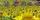Cycling race was run at an average speed of 45 km/h. One cyclist lost with defect 8 minutes. How long and how far he must go at speed 51 km/h to catch peleton again?
7. WavelengthCalculate the wavelength of the tone frequency 11 kHz if the sound travels at speeds of 343 m/s.
8. Cars 6At 9:00 am two cars started from the same town and traveled at a rate of 35 miles per hour and the other car traveled at a rate of 40 miles per hour. After how many hours will the cars be 30 miles apart?
9. StormSo far, a storm has traveled 35 miles in 1/2 hour in direction straight to observer. If it is currently 5:00 p. M. And the storm is 105 miles away from you, at what time will the storm reach you? Explain how you solved the problem.
10. Round-tripA woman works at a law firm in city A which is about 50 miles from city B. She must go to the law library in city B to get a document. Find how long it takes her to drive​ round-trip if she averages 40 mph.
11. Average height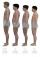In a class are 34 students. The average height of students is 165 cm. What will be the average height of students in the classroom when two pupils tall 176 cm and 170 cm moved from this school/class?
12. Theorem proveWe want to prove the sentence: If the natural number n is divisible by six, then n is divisible by three. From what assumption we started?
13. AverageIf the average(arithmetic mean) of three numbers x,y,z is 50. What is the average of there numbers (3x +10), (3y +10), (3z+10) ?
14. Median and modusRadka made 50 throws with a dice. The table saw fit individual dice's wall frequency: Wall Number: 1 2 3 4 5 6 frequency: 8 7 5 11 6 13 Calculate the modus and median of the wall numbers that Radka fell.
15. AverageThe arithmetic mean of the two numbers is 71.7. One number is 5. Calculate the second number.
16. 75th percentile (quartille Q3)Find 75th percentile for 30,42,42,46,46,46,50,50,54
17. Freight and passenger car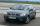The truck starts at 8 pm at 30 km/h. Passenger car starts at 8 pm at 40 km/h. Passenger arrives in the destination city 1 hour and 45 min earlier. What is the distance between the city of departure and destination city?